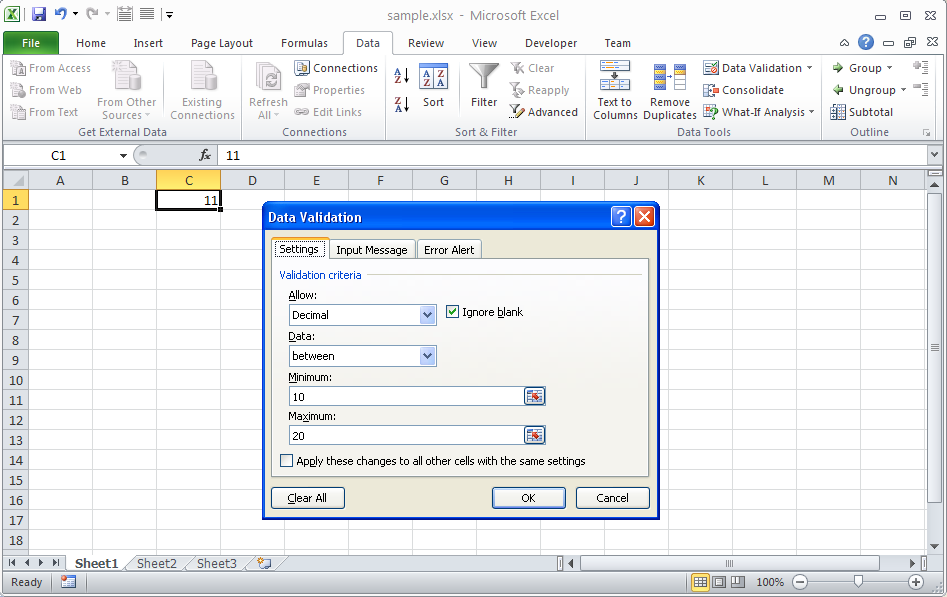# Verify that Cell Value Satisfies Data Validation Rules

Contents
[ ]

## Verify that Cell Value Satisfies Data Validation Rules

Sometimes, it is necessary to dynamically verify if a given value satisfies the data validation rules applied to the cell. For this purpose, the Aspose.Cells APIs provide the cell.getValidationValue method. If the value in a cell does not satisfy the data validation rule applied to that cell, it returns False, else True.

The following sample Microsoft Excel file is used with the sample code below to test the cell.getValidationValue method. As you can see in the snapshot that the cells C1 has decimal data validation applied and will only accept values between 10 and 20. Whenever the value of the cell is between 10 and 20, cell.getValidationValue method will return True, otherwise, it will return False.The following sample code illustrates how the cell.getValidationValue method works. First, it enters the value 3 into C1. Because this does not satisfy the data validation rule, the cell.getValidationValue method returns False. Then, it enters the value 15 into C1. Because this value satisfies the data validation rule, the cell.getValidationValue method returns True. Similarly, it returns False for value 30.

## Console output generated by the sample code

Here is the console output generated when the sample code is executed with the sample Excel file shown above.

``````Is 3 a Valid Value for this Cell: False

Is 15 a Valid Value for this Cell: True

Is 30 a Valid Value for this Cell: False``````